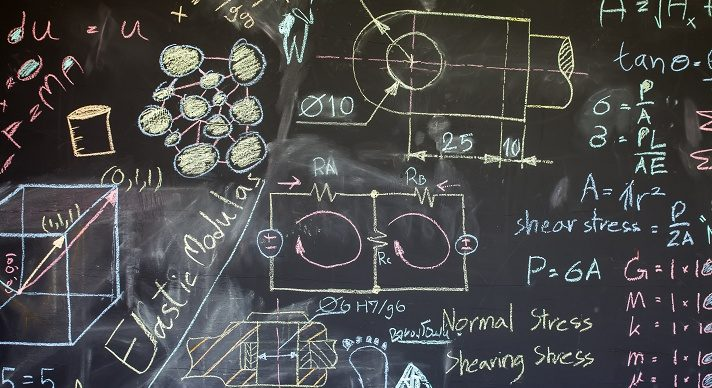# AP Calculus BC### Course Overview

Acellus AP Calculus is a two-part advanced placement course providing students with the curriculum required by the College Board for AP Calculus AB and BC. Students completing this course will be able to take the AP Calculus exam, enabling them to earn college credit for taking this course while still in high school. Besides learning how to use the basic tools of Calculus, students completing this course learn on a deeper level what they are really doing and why it works. This provides insight few students experience in more conventional Calculus courses, empowering them with the knowledge required to solve real world problems. Acellus AP Calculus BC is taught by veteran AP Calculus teacher, Patrick Mara.  This course has been audited and approved by the College Board. Acellus AP Calculus BC is A-G Approved through the University of California.

### Course Objectives & Student Learning Outcomes

Acellus AP Calculus is a two part-series consisting of Acellus AP Calculus AB and BC. Students completing this series will have taken the equivalent of two-semesters of college Calculus. (These courses have been audited and approved by the College Board to carry the AP designation and have been acknowledged to provide students with a college-level learning experience.) Students successfully completing these courses will know what limits are and how to compute them. They know how to differentiate functions and use various differentiation rules including the combination rules and the chain rule. They know how to use Calculus to analyze various functions and sketch graphs based on their derivatives. They are familiar using derivatives in real world situations. Students know how to approximate the area under curves using numerical methods and how to calculate the exact area using integration. Students are familiar with various integration techniques, including the chain rule, u-substitution, integration by parts, trig substitution, and partial fractions. They know how to use integration to calculate volumes of solids of revolution and surface area. Students know how to use integration to solve various real world problems including work problems and problems based upon liquid pressure and fluid force. They also know how to use separable differential equations and are familiar with slope fields. Students are familiar with sequences and various series and converge tests. Students also know how to work with parametric equations, polar coordinates and functions, and vector quantities and know how to calculate dot and cross products.This course was developed by the International Academy of Science. Learn More

### Scope and Sequence

Unit 1 – Techniques of Integration This unit includes the chain rule, u-substitution, expanding, separating the numerator, completing the square, dividing, adding and subtracting terms, trig identities, integration by parts, trig integrals, trig substitution, partial fractions, L’Hopital’s Rule, improper integrals, and Euler’s Method. This unit is followed by the Mid-Term Review and Exam. Unit 2 – Sequences and Series This unit discusses sequences, telescoping series, integral test and p-series, and alternating series, direct and limit comparison tests, ratio and root tests, Taylor Polynomials and Taylor’s Theorem, power series, and Taylor series for familiar functions. Unit 3 – Parametric Equations This unit covers derivatives and second derivatives of parametric equations, arc length, polar coordinates including area and arc length, and derivatives of polar functions. Unit 4 – Vectors This unit discusses dot and cross products, vector valued functions, differentiation, integrations, velocity, and acceleration. Unit 5 – AP Practice This unit provides practice with problems such as those encountered on the AP Calculus BC exam. This unit is followed by the Final Review and Exam.